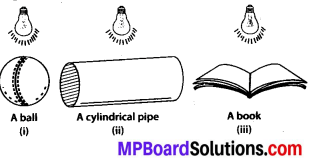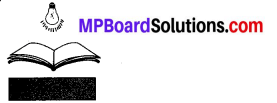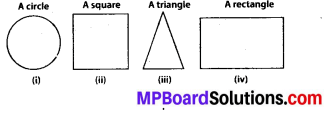# MP Board Class 7th Maths Solutions Chapter 15 Visualising Solid Shapes Ex 15.4

## MP Board Class 7th Maths Solutions Chapter 15 Visualising Solid Shapes Ex 15.4

Question 1.
A bulb is kept burning just right above the following solids. Name the shape of the shadows obtained in each case. Attempt to give a rough sketch of the shadow.Solution:
The shapes of the shadows of these figures will be as follows.
(i) A ballThe shape of the shadow of a ball will be a circle.

(ii) A cylindrical pipeThe shape of the shadow of a cylindrical pipe will be a rectangle.

(iii) A bookThe shape of the shadow of a book will be a rectangle.Question 2.
Here are the shadows of some 3-D objects, when seen under the lamp of an overhead projector. Identify the solid(s) that match each shadow. (There may be multiple answers for these!)Solution:
The given shadows can be obtained in case of the following objects.
(i) Compact disk
(ii) A dice
(iii) Triangular pyramid
(iv) NoteBook

Question 3.
Examine if the following are true statements:
(i) The cube can cast a shadow in the shape of a rectangle.
(ii) The cube can cast a shadow in the shape of a hexagon.
Solution:
A cube can cast a shadow only in the shape of a square. Therefore, any other shape is not possible.